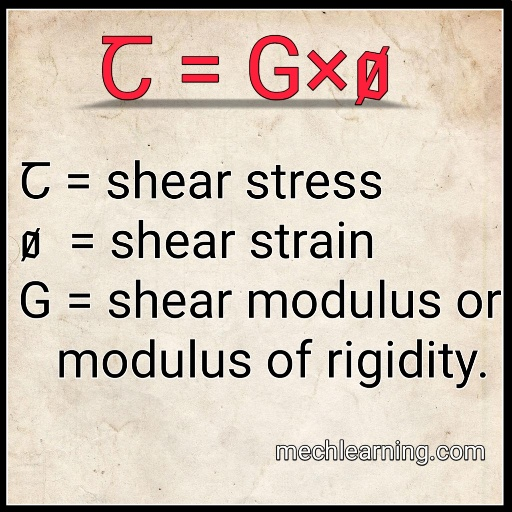# Define strength of material?

Strength of material is The branch of engineering - science which deals with the ability of various types of materials to resist it's fail your and their behaviour by the application of forces.

In strength of material the elastic constants plays the important role for solving problems.
The elastic constants such as Young's modulus or modulus of elasticity, modulus of rigidity, bulk modulus etc.

## What is Young Modulus, Modulus of Rigidity,  Bulk Modulus and Poisson’s Ratio - definitions, formulas and units.

### Young's modulus or modulus of elasticity:-Young's modulus or modulus of elasticity

The hooks law state that when the material is loaded within the elastic limit the stress is directly proportional to the strain.

To remove the proportionality sign we use a constant known as Young's modulus or modulus of elasticity.
The modulus of elasticity or Young's modulus is denoted by letter E.

Stress = modulus of elasticity or young's modulus  × strain

Mathematically,

σ α ε

σ = E ε

σ = stress
ε = strain
E = modulus of elasticity.
Where E is a constant of proportionality and it is known as Young's modulus for modulus of elasticity.

The unit of modulus of elasticity or Young's modulus is Nm-2 or Pascal.

### Shear modulus or modulus of rigidity:-Shear modulus or modulus of rigidity

A shear modulus is found experimentally that within the elastic limit, the shear stress is directly proportional to the shear strain.

To remove the proportionality sign we use a constant known as shear modulus or modulus of rigidity.
The modulus of rigidity or shear modulus is denoted by letter G.

Shear stress = modulus of rigidity or shear modulus  × Shear strain

Mathematically,

α

= ×

= shear stress
= shear strain
G = shear modulus or modulus of rigidity.

Where G is a constant of proportionality and it is known as shear modulus or modulus of rigidity.
Unit of shear shear modulus or modulus of rigidity is Nm-2 or Pascal.

### Bulk modulus:-

When a body is subjected to three mutually perpendicular stresses that are equal in intensity and the ratio of direct stress to the corresponding volumetric strain is called as bulk modulus.
The bulk modulus is denoted by letter K.

Bulk modulus = direct stress / volumetric strain

Mathematically,

K = σ / εV

K = Bulk modulus
σ = direct stress
εV = volumetric strain.

The unit of modulus of elasticity or Young's modulus is Nm-2 or Pascal.

### Poisson’s Ratio:-Poison's ratio

The poison's ratio is defined as the ratio of lateral strain to the linear strain. This ratio called as poison's ratio.
The poison's ratio denoted by Greek letter μ.

Poison's ratio = lateral strain (ε)/ linear strain (ε)

Mathematically,

μ = ε (lateral) / ε (linear)

μ = poison's ratio

Poisons ratio has no units.

Here we concluding, If you have any queries about the topic of  What is Young Modulus, Modulus of Rigidity,  Bulk Modulus and Poisson’s Ratio - definitions, formulas and units then feel free to comment below.

Related articles:

Previous Post
Next Post

#### Mech Learning

##### Related Posts

1.1.